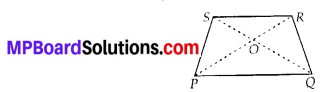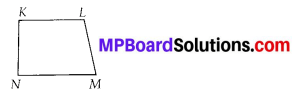# MP Board Class 6th Maths Solutions Chapter 4 Basic Geometrical Ideas Ex 4.5

## MP Board Class 6th Maths Solutions Chapter 4 Basic Geometrical Ideas Ex 4.5

Question 1.
Draw a rough sketch of a quadrilateral PQRS. Draw its diagonals. Name them. Is the meeting point of the diagonals in the interior or exterior of the quadrilateral?
Solution:Diagonal PR and diagonal SQ meet at point O, which is in the interior of the quadrilateral PQRS.Question 2.
Draw a rough sketch of a quadrilateral KLMN. State,
(a) two pairs of opposite sides,
(b) two pairs of opposite angles,
Solution:(a) Two pairs of opposite sides :
$$\overline{K L}$$ and $$\overline{N M}$$, $$\overline{K N}$$ and $$\overline{L M}$$

(b) Two pairs of opposite angles :
∠K and ∠M, ∠L and ∠N

(c) Two pairs of adjacent sides :
$$\overline{K N}$$ and $$\overline{N M}$$ , $$\overline{K L}$$ and $$\overline{L M}$$

(d) Two pairs of adjacent angles :
∠K and ∠N, ∠L and ∠MQuestion 3.
Investigate :
Use strips and fasteners to make a triangle and a quadrilateral.
Try to push inward at any one vertex of the triangle. Do the same to the quadrilateral.
Is the triangle distorted? Is the quadrilateral distorted? Is the triangle rigid?
Why is it that structures like electric towers make use of triangular shapes and not quadrilaterals?
Solution:
No, the triangle is not distorted but the quadrilateral is distorted and also the triangle is rigid.
Structures like electric towers make use of triangular shape so that they could not be distorted and they could be rigid.